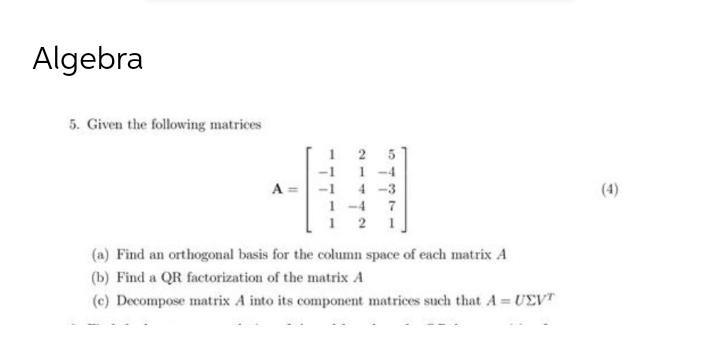Question:

# 5. Given the following matrices 1 2 5 -1 1 -4 A= -1 4 -3 1 -4 7 1 25. Given the following matrices 1 2 5 -1 1 -4 A= -1 4 -3 1 -4 7 1 2 1 (a) Find an orthogonal basis for the column space of each matrix A (b) Find a QR factorization of the matrix A (c) Decompose matrix A into its component matrices such that A=UΣVᵗ